Courses

# Optical Instruments and Scattering of Light Class 12 Notes | EduRev

## Class 12 : Optical Instruments and Scattering of Light Class 12 Notes | EduRev

The document Optical Instruments and Scattering of Light Class 12 Notes | EduRev is a part of the Class 12 Course Physics Class 12.
All you need of Class 12 at this link: Class 12

Human Eye

Human eye is an optical instrument which forms real image of the objects on retina.

Retina colours contains lakhs of cone and rod cells which of light and intensities of light respectively.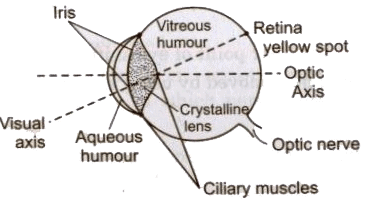Ciliary muscles change the focal length of eye lens. This power of eye is called power of accommodation of eye.

Different defects of vision of human eye are described below

(i) Myopia or Short-Sightedness It is a defect of eye due to which a person can see near by objects clearly but cannot see far away objects clearly.

In this defect, the far point of eye shifts from infinity to a nearer distance.

This defect can be removed by using a concave lens of appropriate power.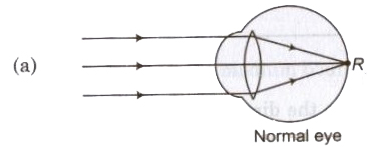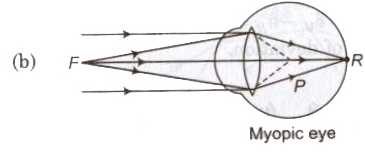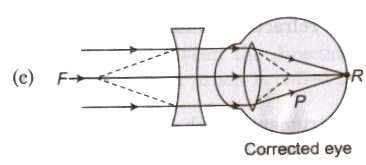(ii) Hypermetropia or Long-Sightedness In this defect, a person can see far away objects clearly but cannot see near by objects clearly.

In this defect the near point of eye shifts away from the eye.

This defect can be removed by using a convex lens of appropriate power.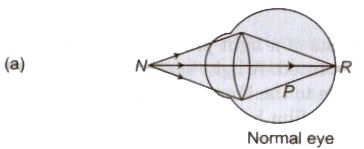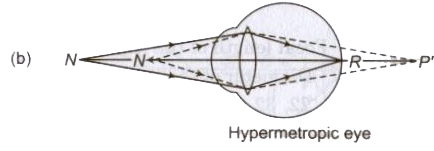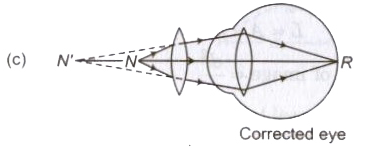(iii) Astigmatism- In this defect, a person cannot focus on horizontal and vertical lines at the same distance at the same time.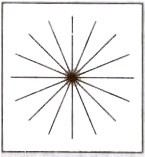This defect can be removed by using suitable cylindrical lenses.

(iv) Colour Blindness In this defect, distinguish between few colours. a person is unable to The reason of this defect is the absence few colours. of cone cells sensitive for

This defect cannot be removed.

(v) Cataract In this defect. an opaque white membrane is developed on cornea due to which person lost power of vision partially on completely.

This defect can be removed by removing this membrane through surgery.

Camera

A photograph camera consists of a light proof box, at one end of which a converging lens system is fitted. A light sensitive film is fixed at the other end of the box, opposite to the lens system. A real inverted image of the object is formed on the film by the lens system.

f-Number for a Camera: The f-number represent the size of the aperture.

f-number =Focal length of the lens (F) / Diameter of the lens(d)

Generally 2, 2.8, 4, 5.6, 8, 11, 22, 32 are f-numbers.

The amount of light (L) entering the camera is directly proportional to the area (A) of the aperture, i.e.,

L ∝A∝ d2

Brightness of Image ∝ (d2/f2)

where, d = diameter of the lens and F = focal length of the lens.

Exposure time is the time for which light is incident of photographic film.

Simple Microscope

It is used for observing magnified images of objects. It is consists of a converging lens of small focal length.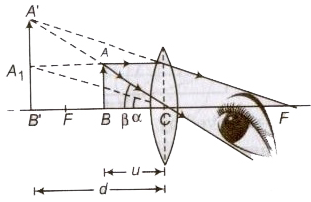Magnifying Power

(i) When final image is formed at least distance of distinct vision (D), then M=1+d/f

where, f= focal length of the lens.

(ii) When final image is formed at infinity, then M = D/f

Compound Microscope

It is a combination of two convex lenses called objective lens and eye piece separated by a distance. Both lenses are of small focal lengths but fo < fe, where fo and fe are focal lengths of objective lens and eye piece respectively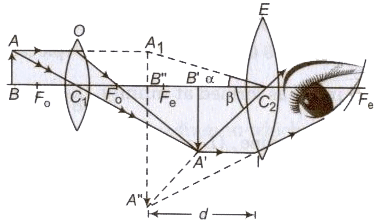Magnifying Power

M = vo / uo {1 + (D/fo)

Where vo= distance of image, formed by objective lens and
uo = distance of object from the objective

(ii) When final image is formed at infinity, then
M = vo/uo . D/fe

Astronomical Telescope

It is also a combination of two lenses, called objective lens and eye piece, separated by a distance. It is used for observing distinct images of heavenly bodies like stars, planets etc.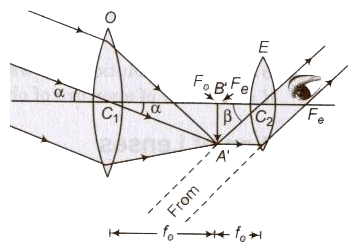Magnifying Power

(i) When final image is formed at least distance of distinct vision (D), then M = fo/fe {1+ (D/fe)} where fo and fe are focal lengths of objective and eyepiece respectively.

Length of the telescope (L) = (fo + ue)

where, ue = distance of object from the eyepiece.

(ii) When final image is formed at infinity, then M = fo/fe

Length of the telescope (L) = fo + fe

For large magnifying power of a telescope fo should be large and fe should be small.

For large magnifying power of a microscope; fo < fe should be small.

Resolving Power

The ability of an optical instrument to produce separate and clear images of two near by objects, is called its resolving power.

Limit of Resolution

The minimum distance between two near by objects which can be just resolved by the instrument, is called its limit of resolution (d).

Resolving power of a microscope = 1/d = 2 μ sin θ / λ

where, d = limit of resolution, λ = wavelength of light used.
μ = refractive index of the medium between the objects and objective lens and θ = half of the cone angle.

Resolving power of a telescope = 1/dθ = d/1.22 λ

where, dθ = limit of resolution, A = wavelength of light used and
d = diameter of aperture of objective

Aberration of Lenses

The image formed by the lens suffer from following two main drawbacks:-

(i) Spherical Aberration-Aberration of the lens due to which the rays passes through the lens are not focussed at a single and the image of a point object placed on the axis is blurred. called spherical aberration.

It can be reduced by using

• lens of large focal lengths
• plano-convex lenses
• crossed lenses
• combining convex and concave lens

(ii) Chromatic Aberration Image of a white object formed by lens is usually coloured and blurred. This defect of the image produced by lens is called chromatic aberration.

Scattering of Light

When light passes through a medium in which particles are suspended whose size is of the order of wavelength of light, then light on striking these particles, deviated in different directions. These phenomena is called scattering of light.

According to the Lord Rayleigh, the intensity of scattered light

I ∝ 1/λ4

Therefore, red colour of light is scattered least and violet colour of light is scattered most.

Daily Life Examples of Scattering of Light

1. Blue colour of sky.
2. Red colour of signals of danger.
3. Black colour of sky in the absence of atmosphere
4. Red colour of the time of sun rise and sun set.
5. The human eye is most sensitive to yellow colour
Offer running on EduRev: Apply code STAYHOME200 to get INR 200 off on our premium plan EduRev Infinity!

,

,

,

,

,

,

,

,

,

,

,

,

,

,

,

,

,

,

,

,

,

;